# leetcode刷题（75）— 547. 朋友圈

输入:
[[1,1,0],
[1,1,0],
[0,0,1]]



输入:
[[1,1,0],
[1,1,1],
[0,1,1]]



N 在[1,200]的范围内。

Union-Find 算法，也就是常说的并查集算法，主要是解决图论中「动态连通性」问题的。

class UF {
/* 将 p 和 q 连接 */
public void union(int p, int q);
/* 判断 p 和 q 是否连通 */
public boolean connected(int p, int q);
/* 返回图中有多少个连通分量 */
public int count();
}


1、自反性：节点p和p是连通的。

2、对称性：如果节点p和q连通，那么q和p也连通。

3、传递性：如果节点p和q连通，q和r连通，那么p和r也连通。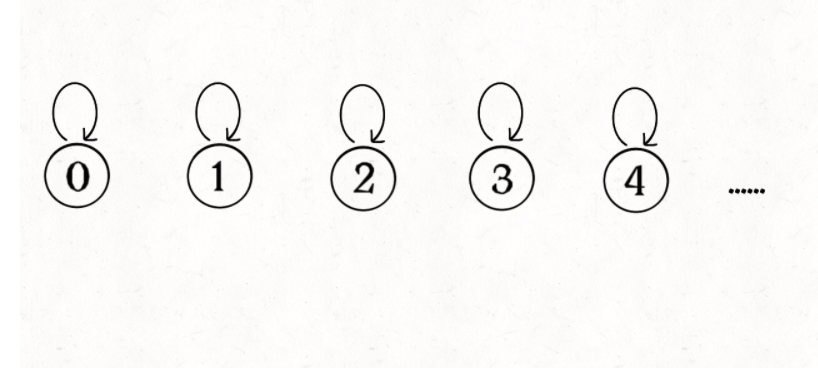class UF {
// 记录连通分量
private int count;
// 节点 x 的节点是 parent[x]
private int[] parent;

/* 构造函数，n 为图的节点总数 */
public UF(int n) {
// 一开始互不连通
this.count = n;
// 父节点指针初始指向自己
parent = new int[n];
for (int i = 0; i < n; i++)
parent[i] = i;
}

/* 其他函数 */
}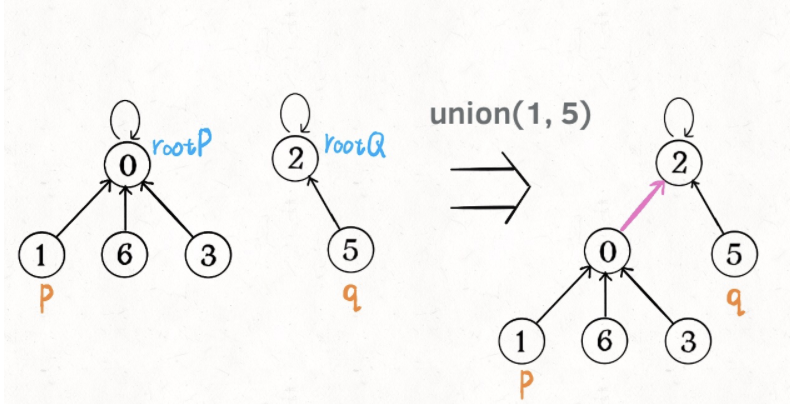public void union(int p, int q) {
int rootP = find(p);
int rootQ = find(q);
if (rootP == rootQ)
return;
// 将两棵树合并为一棵
parent[rootP] = rootQ;
// parent[rootQ] = rootP 也一样
count--; // 两个分量合二为一
}

/* 返回某个节点 x 的根节点 */
private int find(int x) {
// 根节点的 parent[x] == x
while (parent[x] != x)
x = parent[x];
return x;
}

/* 返回当前的连通分量个数 */
public int count() {
return count;
}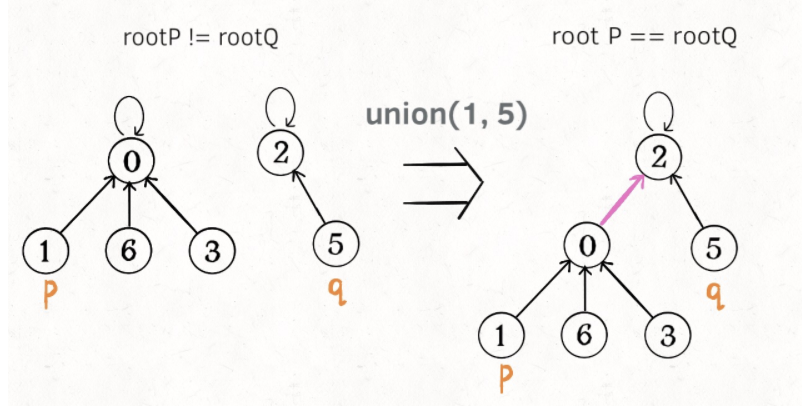public boolean connected(int p, int q) {
int rootP = find(p);
int rootQ = find(q);
return rootP == rootQ;
}


find主要功能就是从某个节点向上遍历到树根，其时间复杂度就是树的高度。我们可能习惯性地认为树的高度就是logN，但这并不一定。logN的高度只存在于平衡二叉树，对于一般的树可能出现极端不平衡的情况，使得「树」几乎退化成「链表」，树的高度最坏情况下可能变成N。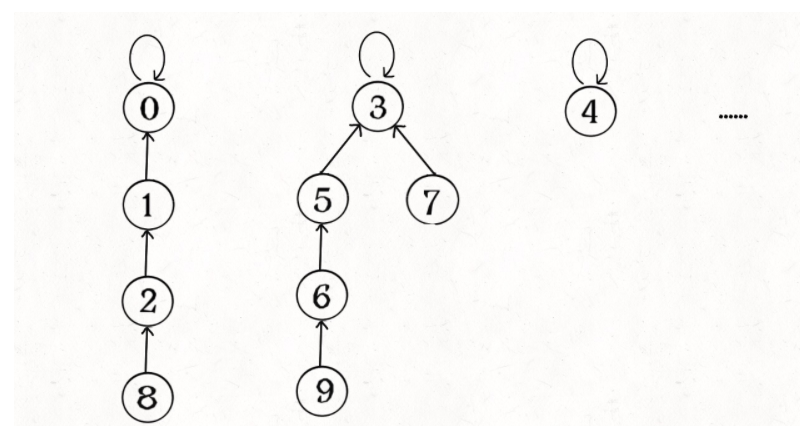public void union(int p, int q) {
int rootP = find(p);
int rootQ = find(q);
if (rootP == rootQ)
return;
// 将两棵树合并为一棵
parent[rootP] = rootQ;
// parent[rootQ] = rootP 也可以
count--;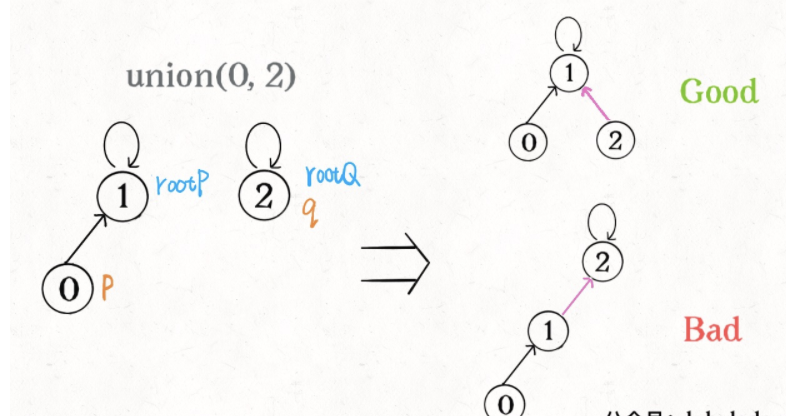class UF {
private int count;
private int[] parent;
// 新增一个数组记录树的“重量”
private int[] size;

public UF(int n) {
this.count = n;
parent = new int[n];
// 最初每棵树只有一个节点
// 重量应该初始化 1
size = new int[n];
for (int i = 0; i < n; i++) {
parent[i] = i;
size[i] = 1;
}
}
/* 其他函数 */
}


public void union(int p, int q) {
int rootP = find(p);
int rootQ = find(q);
if (rootP == rootQ)
return;

// 小树接到大树下面，较平衡
if (size[rootP] > size[rootQ]) {
parent[rootQ] = rootP;
size[rootP] += size[rootQ];
} else {
parent[rootP] = rootQ;
size[rootQ] += size[rootP];
}
count--;
}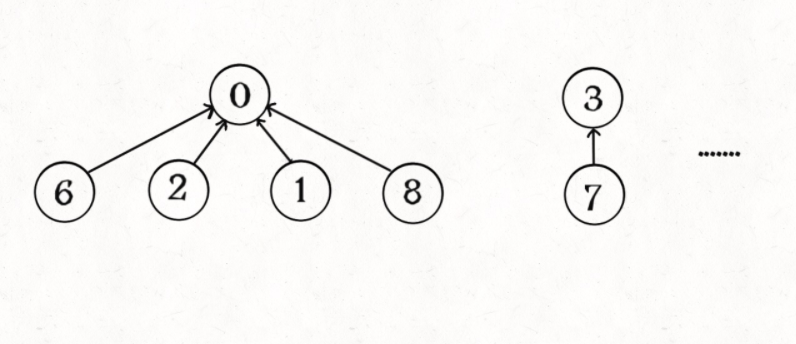private int find(int x) {
while (parent[x] != x) {
// 进行路径压缩
parent[x] = parent[parent[x]];
x = parent[x];
}
return x;
}



class Solution {
public int findCircleNum(int[][] M) {
int len = M.length;
UF uf = new UF(len);
for (int i = 0; i < len; i++) {
for (int j = 0; j < i; j++) {
if (M[i][j] == 1) {
uf.union(i, j);
}
}
}
return uf.getCount();
}
}

class UF {
// 存储一棵树
private int[] parent;
// 记录树的“重量”
private int[] size;
// 连通分量个数
private int count;

UF(int n) {
parent = new int[n];
size = new int[n];
for (int i = 0; i < n; i++) {
parent[i] = i;
size[i] = 1;
}
count = n;
}

public boolean isConnected(int p, int q) {
int rootP = findRoot(p);
int rootQ = findRoot(q);
return rootP == rootQ;
}

private int findRoot(int x) {
while (parent[x] != x) {
// 进行路径压缩
parent[x] = parent[parent[x]];
x = parent[x];
}
return x;
}

public void union(int p, int q) {
int rootP = findRoot(p);
int rootQ = findRoot(q);
if (rootP == rootQ) {
return;
}
int sizeP = size[p];
int sizeQ = size[q];
// 小树接到大树下面，较平衡
if (sizeP > sizeQ) {
parent[rootQ] = rootP;
size[rootP] += size[rootP];
} else {
parent[rootP] = rootQ;
size[rootQ] += size[rootP];
}
count--;
}

public int getCount() {
return count;
}
}


06-15261

#### LeetCode第547题 朋友圈 Friend Circles -- 超详细解析10-24238

#### 【leetcode】547 朋友圈（图，DFS，BFS，并查集）

04-2595

#### leetcode 547.朋友圈

08-31336

#### day 25-26 算法：并查集、岛屿的个数、朋友圈问题

02-061万+

#### LeetCode算法题集-547. Friend Circles（朋友圈）

02-0882

#### leetcode 547. 朋友圈

06-0993

#### leetcode547. 朋友圈©️2020 CSDN 皮肤主题: 大白 设计师: CSDN官方博客点击重新获取扫码支付1.余额是钱包充值的虚拟货币，按照1:1的比例进行支付金额的抵扣。
2.余额无法直接购买下载，可以购买VIP、C币套餐、付费专栏及课程。余额充值